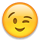# Is it possible to filter an instance on a circular shape ?

Hello,
I want to remove instances which do not overlap with a circular form using a Python script.
For sure, Klayout gives the possibility to check the overlap betwwen two boxes, but I don't see how to check the overlap between a box and a circular form.

``````box=....
if not box.overlaps(db.Box(inst.bbox())):
inst.delete()
``````

Thank you.

Regards,

• @ahmedo

This will find all boxes outside of a layer/circle
Run the script and you will see a circle with boxes inside,
click undo and you can see what has been removed

``````# Enter your Python code here
import pya
import array
import sys
import math
import time

mw = pya.Application.instance().main_window()
layout = pya.Application.instance().main_window().current_view().active_cellview().layout()
topcell = pya.Application.instance().main_window().current_view().active_cellview().cell
lv = pya.Application.instance().main_window().current_view()

if layout == None:
raise Exception("No layout")

cv = pya.Application.instance().main_window().current_view().active_cellview()

dbu =layout.dbu

cell = pya.Application.instance().main_window().current_view().active_cellview().cell
if cell == None:
raise Exception("No cell")

n =   2000 # number of points
da = 2 * math.pi / n

def coords(path):
result = []
for p in path:
result.append(DPoint.new(p/dbu,p/dbu))
return result

def create_polygon(crd,lay):
layer_info = LayerInfo.new(lay,0)
layer_index = layout.layer(layer_info)
cv.cell.shapes(layer_index).insert(Polygon.new(crd))

def create_box(crd,lay):
layer_info = LayerInfo.new(lay,0)
layer_index = layout.layer(layer_info)
cv.cell.shapes(layer_index).insert(Box.new(crd,crd))

circle = []
for i in range(0, n):
path = r * math.cos(i * da), r * math.sin(i * da)
circle.append(path)

lay1 = 9
lay2 = 5

pth = coords(circle)
create_polygon(pth,lay1)

box = [(-6.818,-1.185), (-5.195,3.591)]
crd = coords(box)
create_box(crd,lay2)

box = [(5.742,-2.333), (7.674,2.771)]
crd = coords(box)
create_box(crd,lay2)

box = [(-2.827,0.849), (-1.326,2.469)]
crd = coords(box)
create_box(crd,lay2)

box = [(0.730,-2.708), (2.231,-1.088)]
crd = coords(box)
create_box(crd,lay2)

def cut_region(lay1,lay2):
lv.transaction("Undo")

lif1 = LayerInfo.new(lay1,0)
li1 = layout.layer(lif1)

lif2 = LayerInfo.new(lay2,0)
li2 = layout.layer(lif2)
r1 = pya.Region(topcell.begin_shapes_rec(li1))
r2 = pya.Region(topcell.begin_shapes_rec(li2))

#Boolean Functions
#outside  = r1 -  r2
#outside  = r2 - r1
#outside  = r2 + r1
#outside  = r1 + r2
#outside  = r1 & r2
outside  = r2 & r1
#outside  = r2 ^ r1
#outside  = r1 ^ r2

layout.clear_layer(li2)
output = layout.layer(lif2)
topcell.shapes(output).insert(outside)

lay1 = 9
lay2 = 5
cut_region(lay1,lay2)

lv.commit()

``````
• If I understand @ahmedo correctly he said not overlapping, so instead of
`outside = r2 & r1`
it should be
`not_outside = r2.select_not_outside(r1)`
and then
`topcell.shapes(output).insert(not_outside)`

`r1 & r2` will cut parts of boxes that are outside of the circle.

• @sebastian

I may have misread .. but good point and thanks for highlighting this, we all learn

• No worries ^^. I also think overlapping is not clearly defined in daily speech, so you may very well be correct. Regions were confusing when I started with KLayout, so I wanted to point out that there are fancy options in the regions included that might save a lot of time.

• Thank you @tagger5896 and @sebastian for your valuable help.

I will make a test with your code. I see that the code looks for the name of the layout at the beginning, so I guess I need to run the script using the command line:
`klayout -r <script.py> my.gds`

Is it right ?

By the way I have implemented a solution that allows me to check the overlap:

``````filter_poly = db.Polygon(circular_shape.polygon)
for inst in layout_insts:
# Define the 4 points of the instance box
llp = db.Point(inst.bbox().left,inst.bbox().bottom)
lrp = db.Point(inst.bbox().right,inst.bbox().bottom)
tlp = db.Point(inst.bbox().left,inst.bbox().top)
trp = db.Point(inst.bbox().right,inst.bbox().top)

let = 0
if filter_poly.inside(llp) or filter_poly.inside(lrp) or filter_poly.inside(tlp) or filter_poly.inside(trp):
let = 1

# if let = 0 , then remove instance.
if let == 0 :
inst.delete()
``````

Regards

• For my question I think that I have undestood that @tagger5896 's code should be executed from macro menuI'm used to creating scripts that I execute always in batch mode.

Regards

• @ahmedo
You should have an open layout and run the code from the Macro IDE menu yes.

• @tagger5896

Thank you so much for your help.

Regards

• @sebastian
thanks for pointing this out ... floodgates open and the sea's part,
and yes this makes it easiernot_outside = r2.select_not_outside(r1)